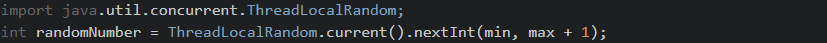Java - Generating random integers within a specific rangeJava, random

While generating random int values in a specific range is fairly simple, it still requires that you understand the syntax and how to use the Java Random class. Let's take a quick look at some examples below which go over some options when generating a random number in Java depending on your Java version.

Java 1.6 and older

In older version of java it is pretty standard to use the java.util.Random class. This is preferable to using the java.lang.Math.random() because why reinvent the wheel? Use the standard library!

import java.util.Random;
public static int randomInteger(int min, int max) {
Random rand;
int randomNumber = rand.nextInt((max-min) + 1) + min;
return randomNumber;
}

Java 1.7 and newer

In newer versions of java we have the advantage of not having to explicitly instantiate the java.util.Random class. Instead we are able to use the a ThreadLocalRandom, check out the example below.